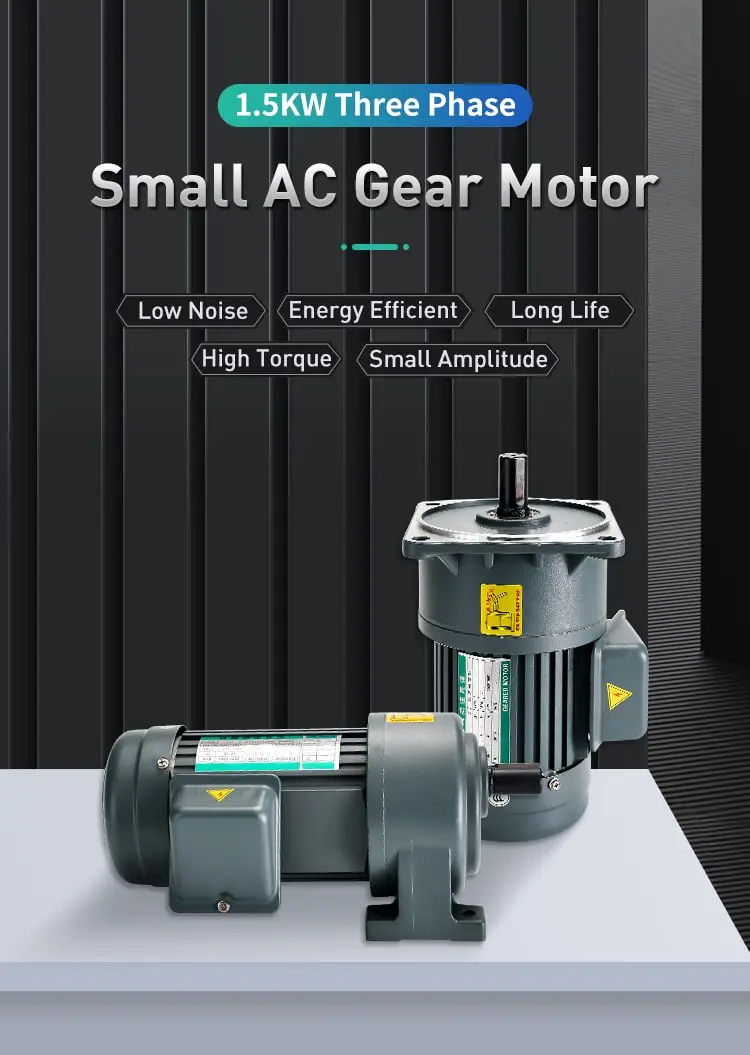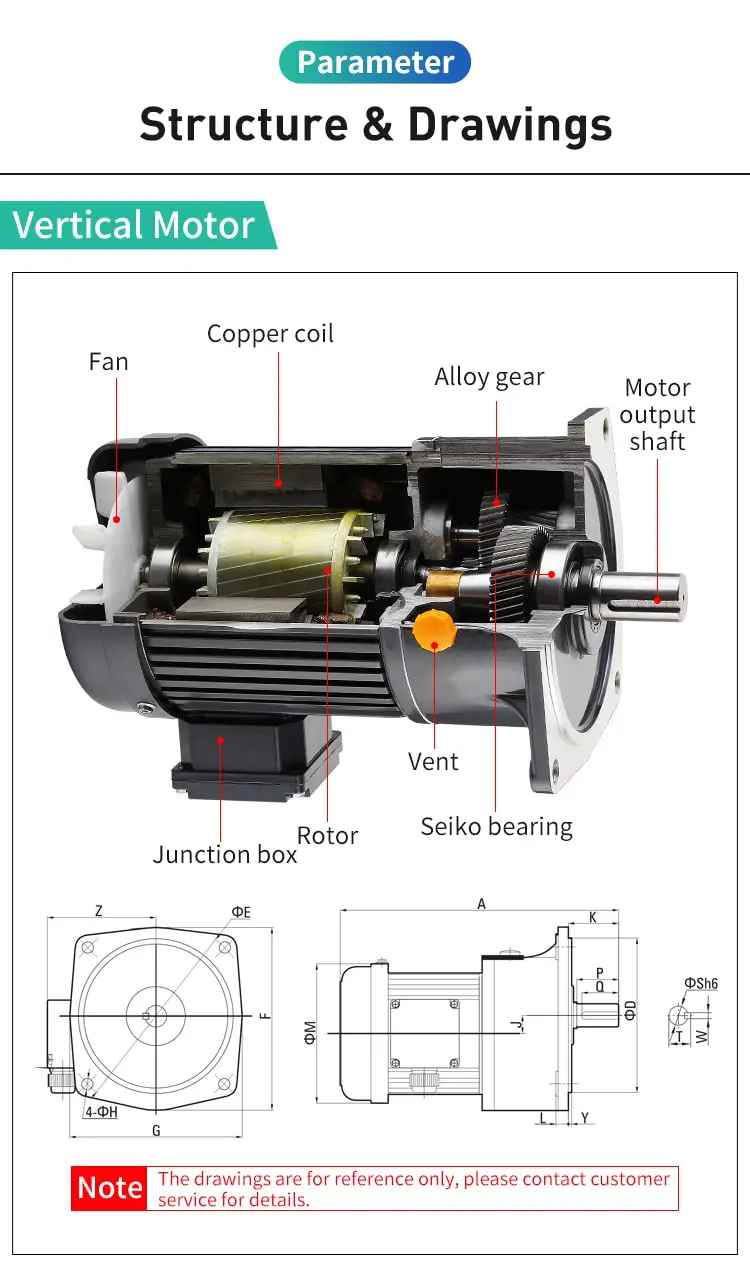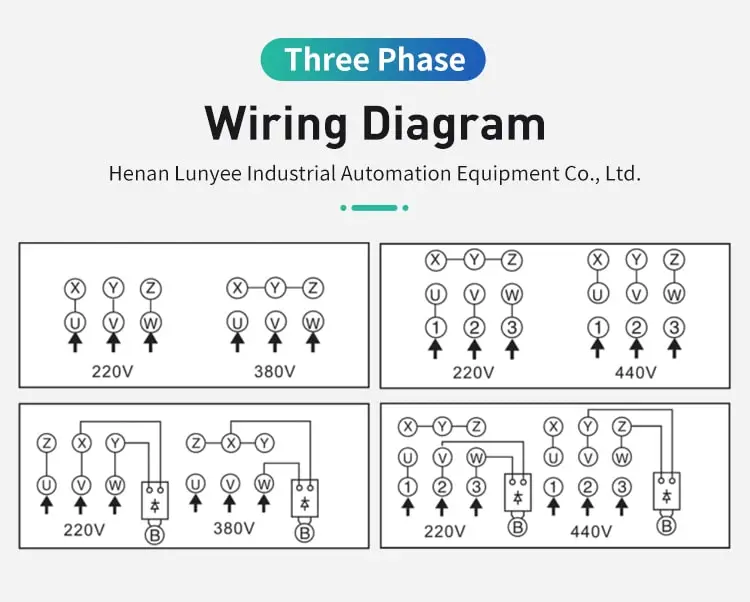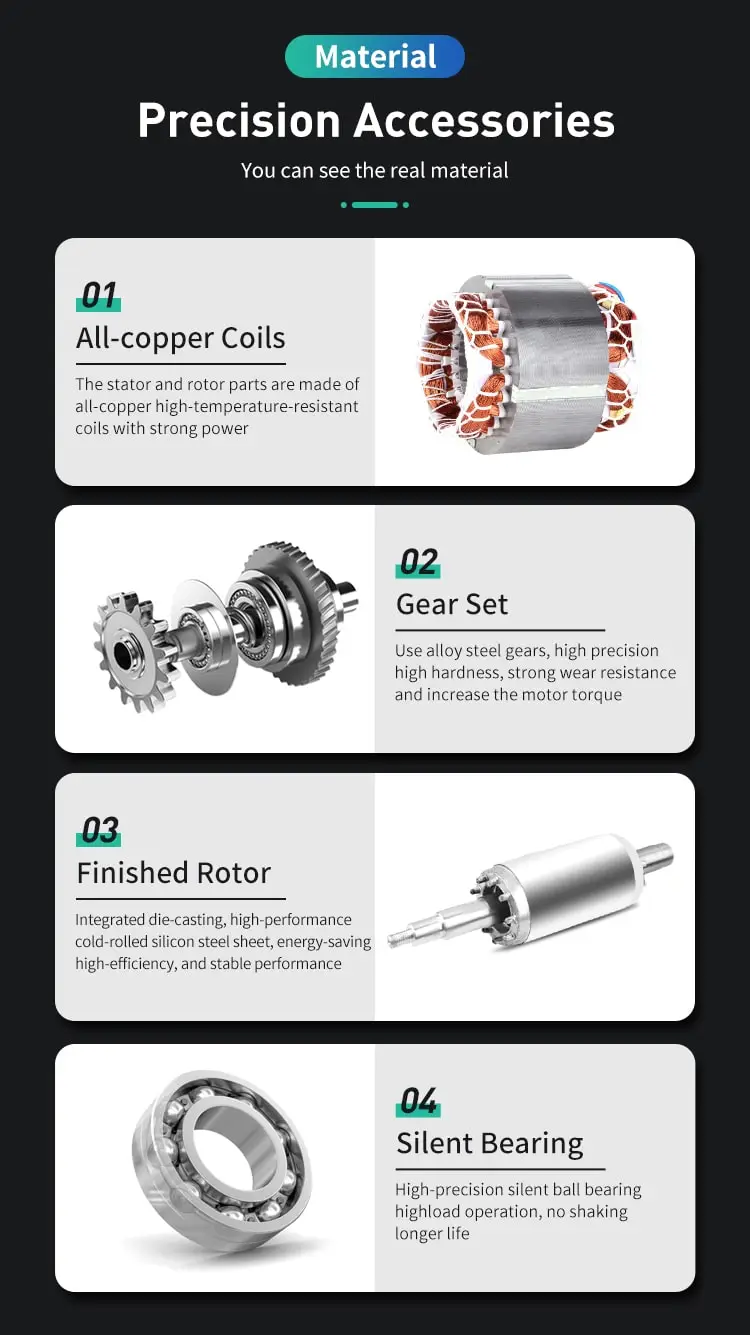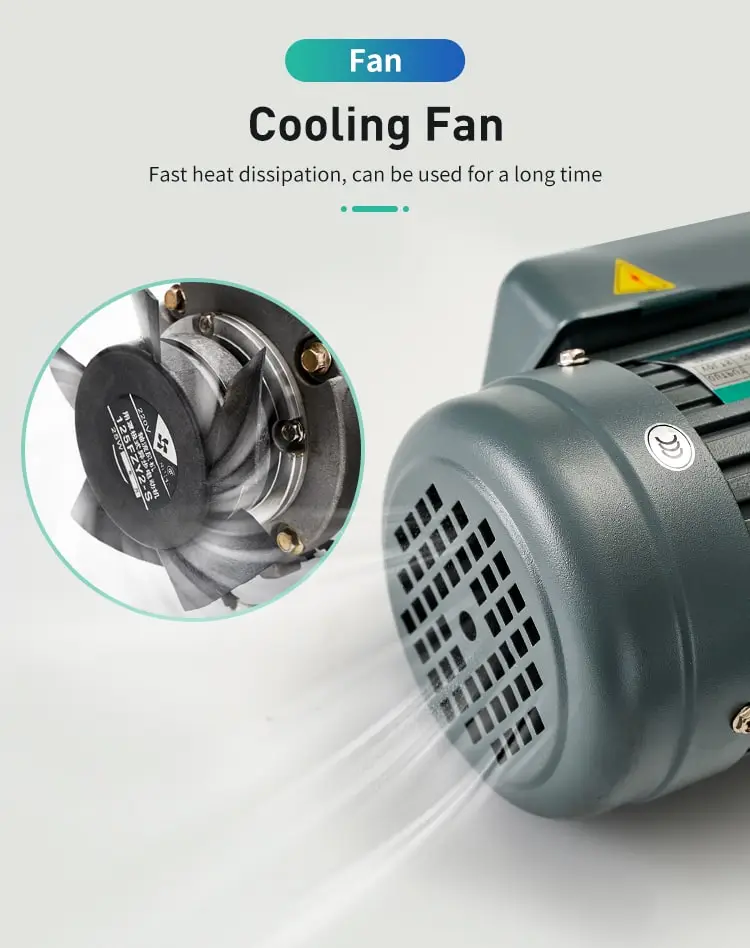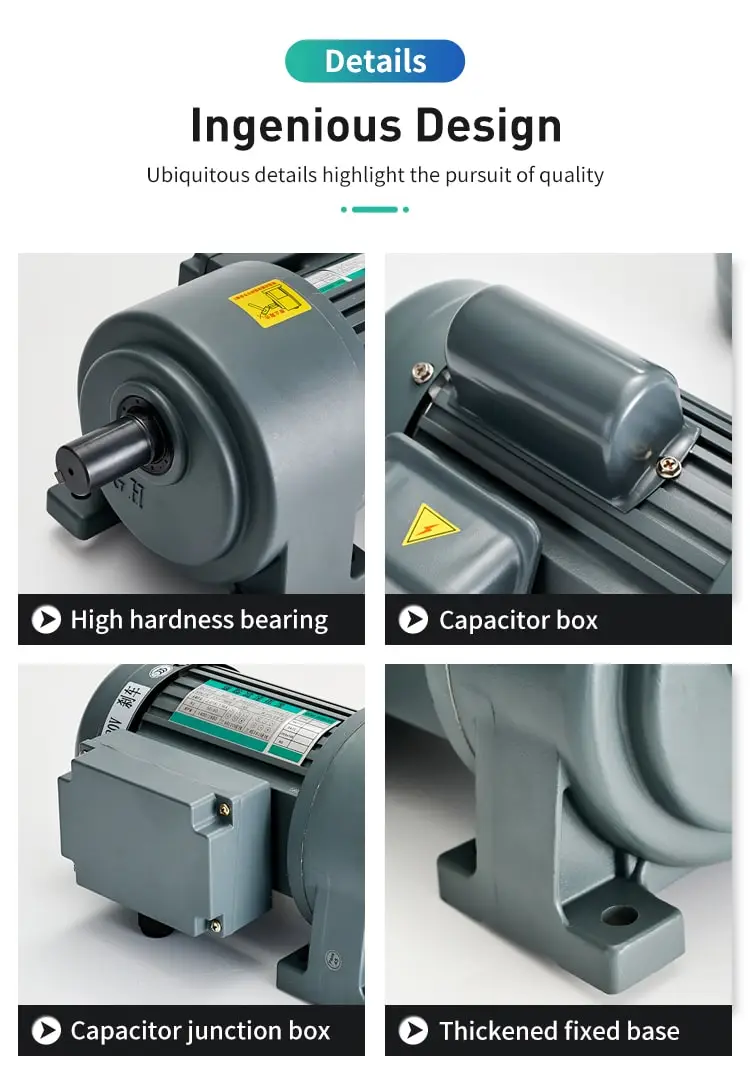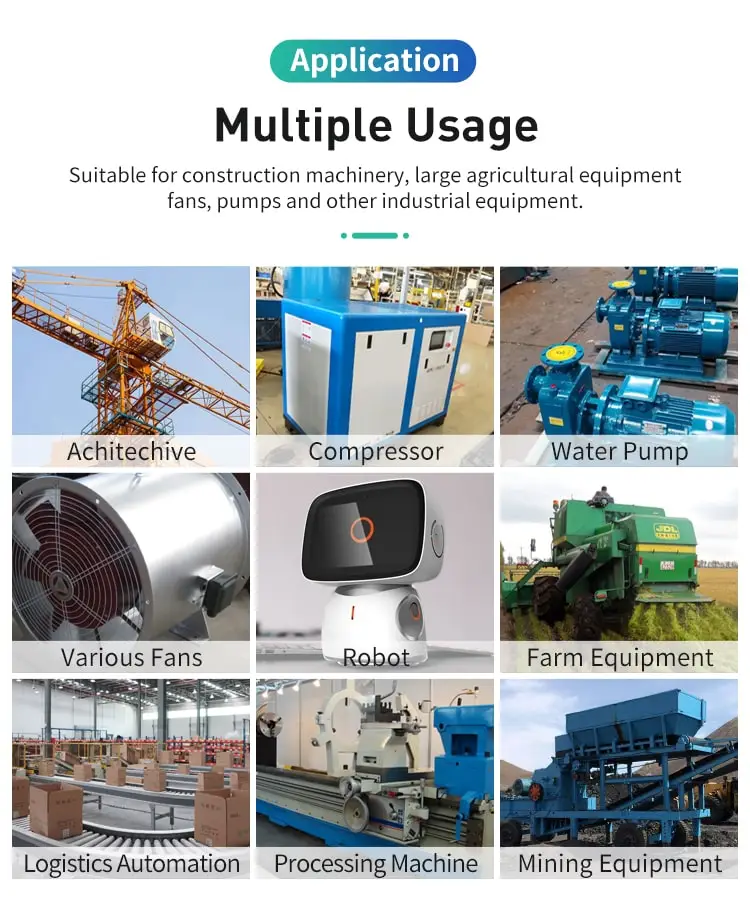By Olivia 2023-01-04 15:39:58

#### What determines the speed of an ac induction motor?

The speed of an AC induction motor is governed by the frequency of the AC power supply and the number of poles in the motor's structure.

The speed of an AC induction motor is determined by the frequency of the AC power supply and the number of poles in the motor's construction.

AC induction motors operate on the principle of electromagnetic induction, in which a rotating magnetic field is used to generate mechanical torque, or rotational force, in the motor's rotor. The frequency of the AC power supply determines the speed of the rotating magnetic field, and thus the speed of the motor.

The number of poles in the motor's construction, on the other hand, determines the number of times the magnetic field rotates per revolution of the rotor. A two-pole motor, for example, will have a magnetic field that rotates twice per revolution of the rotor, while a four-pole motor will have a magnetic field that rotates four times per revolution of the rotor.

In general, the speed of an AC induction motor can be calculated using the following formula:

Speed (in RPM) = (120 x Frequency) / (Number of Poles)

For example, a four-pole AC induction motor operating on a 60 Hz power supply will have a speed of 1,800 RPM (revolutions per minute), while a two-pole motor operating on the same power supply will have a speed of 3,600 RPM.

It is important to note that the speed of an AC Induction motor is not fixed and can vary depending on the load being applied to the motor. When a load is applied to the motor, the rotor will slow down, reducing the speed of the rotating magnetic field and the speed of the motor.

To maximize the efficiency and performance of an AC induction motor, it is important to select a motor with the appropriate number of poles and operating frequency for the intended application. It is also important to properly size the motor for the intended load and to ensure that the motor is operated within its rated capacity.

In conclusion, the speed of an AC induction motor is determined by the frequency of the AC power supply and the number of poles in the motor's construction. By understanding these factors and selecting the appropriate motor for the intended application, it is possible to optimize the performance and efficiency of the motor.